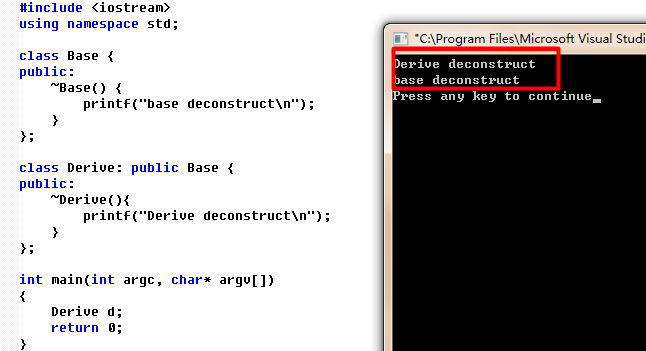6个回答C++作业 派生类构造和析构函数的执行顺序 求大佬帮解答一下

C++ 派生类继承基类的所有成员，这句话对吗？

C++ 派生类继承基类的所有成员，这句话对吗？构造函数、析构函数会被继承吗？求大神们给小弟详细解释下。

C++基类指针怎么调用派生类的方法

![图片说明](https://img-ask.csdn.net/upload/201711/05/1509855025_446289.png)#include <iostream> using namespace std; class Vehicle{ public: stop(){ cout<<"停止"<<endl; system("pause"); } run(){ cout<<"I'm vehicle"<<endl; } }; class Bicycle:public Vehicle{ public: run(){ cout<<"I'm Bicycle"<<endl; } Bicycle(){}; }; class Motorcar:public Vehicle{ public: run(){ cout<<"I'm Motorcar"<<endl; } }; class Motorcycle:public Bicycle,public Motorcar{ public: run(){ cout<<"I'm Motorcycle"<<endl; } }; int main(int argc,char *argv[]){ Vehicle* p; Vehicle veh; Bicycle bic; Motorcar mot; Motorcycle moto; p=&veh; p->run(); p=&bic; p->run(); p=&mot; p->run(); moto.run(); // p=&moto; // p->run(); Vehicle类型指针不能指向moto对象 return 0; }

C++基类派生类继承输出问题！

#include using namespace std; class A{ public: virtual void F(){cout << 1 << endl;} void CallF(){F();} virtual ~A(){CallF(); F();} }; class B : public A{ public: void F(){cout << 2 << endl;} ~B(){} }; class C : public B{ public: void F(){cout << 3 << endl;} void CallF(){F(); A::CallF();} ~C(){CallF();} }; int main(){ A * p = new C(); p->CallF(); delete p; return 0; } 代码输出结果为33311。 我的理解是delete p语句首先调用派生类C析构函数，然后调用基类B的析构函数，接着调用A的析构函数。 当调用C的析构函数时，会执行C类的CallF()函数，为什么这一步会输出两个3？ 当调用A的析构函数，为什么会输出两个1？

1.基类的析构函数不是虚函数，在析构派生类的时候基类会不会被析构 2.基类的析构函数不是虚函数，在析构基类类的时候派生类会不会被析构 3.虚基类的析构函数是不是虚函数？

1.假设有类A,B,C,D,类A是类B的基类，类B是类D的基类，类B有用类C创建的一个对象成员 ，若定义类D的对象，分析调用次序。2.假设类C也从类A派生，即类A同为B和C的基类，其 余同上问，分析。3.对于2问，假设类B和类C 都继承与类A，分析定义类D的对象时， 调用次序

UML图中怎么表达C++中派生类构造函数？

#include<iostream> #include<string> using namespace std; class phone { public: phone() {} phone(string name) { p_name = name; cout << "phone有参构造" << endl; } ~phone() { cout << "phone的析构函数" << endl; } string p_name; }; class student { public: student(string m_name, int m_score, int m_high, string p_name):name(m_name),score(m_score),high(new int(m_high)),pname(m_name){} ~student() { cout << "student的析构函数" << endl; } void pnames() { cout << name << "拿着" << pname.p_name << "手机" << endl; } public: string name; int score; int* high; phone pname; }; int main() { student s; }

c++多选题求大佬帮忙！！！！

#include <iostream> using namespace std; class Base1{ public: Base1(int i){cout<<"Constructing Base1"<<i<<endl; }//父类1有参的构造函数 }; class Base2{ public: Base2(int j){cout<<"Constructing Base2"<<j<<endl; }//父类2有参的构造函数 }; class Base3{ public: Base3(void){cout<<"Constructing Base3 *"<<endl; }//父类3无参的构造函数 }; class Derived:public Base2,public Base1,public Base3//多个基类的继承 { public: Derived{int a,int b,int c,int d}:Base1(a),member2(d),member(c),Base2(b) {}//派生类的构造函数定义 private: Base1 member1; Base2 member2; Base3 member3; }; int main() Derived obj(1,2,3,4);//派生类析构函数的初始化 return 0; }

1、多个类的继承关系画出结构图（五个以上类） 2、定义五个以上类 ，每个类写出类的定义（2个文件） 3、使用静态数据成员和静态成员函数 4、要求有基类和派生类的构造函数 和至少一个析构函数） 5运算符重载 成员函数或友元函数 6、实现多重继承和虚基类 7、使用抽象类，实现多态性 8、主函数

#include <iostream> #include <conio.h> using namespace std; // enum bool {false,true}; struct element { //定义链表中的结点结构 int val; element *next; }; class list { //定义链表类 protected:element *elems; public: list() { elems = 0; } ~list(); virtual bool insert(int); //此虚函数在派生类中可重新定义 virtual bool deletes(int); //此虚函数在派生类中可重新定义 bool contain(int); void print(); }; class set :public list { //将集合类 set 定义为链表类 list 的派生类 int Card; public: set() { Card = 0; } bool insert(int); //重定义此函数 bool deletes(int); //重定义此函数 bool add(set*, set*); bool and(set*, set*); bool minute(set*, set*); friend set operator +(set&, set&); friend set operator -(set&, set&); friend set operator *(set&, set&); }; list::~list() //list 类得析构函数定义，循环释放各元素所占的存储 { element *tmp = elems; for (element *elem = elems; elem != 0;) { tmp = elem; elem = elem->next; delete tmp; } } bool list::insert(int val) //定义 list 类中插入元素的成员函数 { element *elem = new element; //为新元素分配存储 if (elem != 0) { elem->val = val; //将新元素插入到链表头 elem->next = elems; elems = elem; return true; } else return false; } bool list::deletes(int val) //定义 list 类中删除元素的成员函数 { if (elems == 0) return false; //若表为空，返回 false element *tmp = elems; if (elems->val == val) { //若待删除的元素为表头元素 elems = elems->next; delete tmp; return true; } else for (element *elem = elems; elem->next != 0; elem = elem->next) if (elem->next->val == val) { //循环查找待删除元素 tmp = elem->next; elem->next = tmp->next; delete tmp; return true; } return false; } bool list::contain(int val) { //判元素 val 在链表中是否存在，存在返回true，不存在返回false if (elems == 0)return false; if (elems->val == val) return true; else for (element *elem = elems; elem->next != 0; elem = elem->next) if (elem->next->val == val) return true; return false; } void list::print() //输出链表中各元素 { if (elems == 0) return; for (element *elem = elems; elem != 0; elem = elem->next) cout << elem->val << " "; cout << endl; } bool set::insert(int val) //在 set 类中的 insert 的重定义版本 { if (!contain(val)) { //先判断此元素是否存在，然后再调用基类的此函数版本 ++Card; list::insert(val); return true; } return false; } bool set::deletes(int val) //在 set 类中的 deletes 的重定义版本 { if (list::deletes(val)) //调用基类中的此函数版本 { return true; } return false; } bool set::add(set* s1,set* s2) { for (element* elem=s1->elems;elem!=NULL;elem=elem->next) { insert(elem->val); } for (element* elem = s2->elems; elem != NULL; elem = elem->next) { insert(elem->val); } return true; } bool set:: and (set* s1,set* s2) { for (element* elem=s1->elems;elem!=NULL;elem=elem->next) { if (s2->contain(elem->val)) { insert(elem->val); } } return true; } bool set::minute(set* s1,set* s2) { for (element* elem = s1->elems; elem!= NULL; elem = elem->next) { if (!s2->contain(elem->val)) { insert(elem->val); } } return true; } set operator +(set& s1, set& s2) { set s; for (element* elem = s1.elems; elem != NULL; elem = elem->next) { s.insert(elem->val); } for (element* elem = s2.elems; elem != NULL; elem = elem->next) { s.insert(elem->val); } return s; } set operator -(set& s1, set& s2) { set s; set* set1 = &s1; set* set2 = &s2; for (element* elem = set1->elems; elem != NULL; elem = elem->next) { if (!set2->contain(elem->val)) { s.insert(elem->val); } } return s; } set operator *(set& s1, set& s2) { set s; for (element* elem = s1.elems; elem != NULL; elem = elem->next) { if (s2.contain(elem->val)) { s.insert(elem->val); } } return s; } int main() { set *ptr, set1,set2; ptr = &set1; ptr->insert(15); ptr->insert(16); ptr->insert(17); ptr->insert(18); ptr->print(); ptr = &set2; ptr->insert(20); ptr->insert(19); ptr->insert(17); ptr->insert(18); ptr->print(); set set3, set4, set5; ptr = &set3; set3 = (set1 + set2); //set4 = set1 - set2; //set5 = set1 * set2; ptr->print(); ptr->print(); ptr->print(); return 1; }

Java基础知识面试题（2020最新版）

Intellij IDEA 实用插件安利

1. 前言从2020 年 JVM 生态报告解读 可以看出Intellij IDEA 目前已经稳坐 Java IDE 头把交椅。而且统计得出付费用户已经超过了八成（国外统计）。IDEA 的...

MySQL数据库面试题（2020最新版）

2020阿里全球数学大赛：3万名高手、4道题、2天2夜未交卷

HashMap底层实现原理，红黑树，B+树，B树的结构原理 Spring的AOP和IOC是什么？它们常见的使用场景有哪些？Spring事务，事务的属性，传播行为，数据库隔离级别 Spring和SpringMVC，MyBatis以及SpringBoot的注解分别有哪些？SpringMVC的工作原理，SpringBoot框架的优点，MyBatis框架的优点 SpringCould组件有哪些，他们...

《Oracle Java SE编程自学与面试指南》最佳学习路线图2020年最新版（进大厂必备）